# Acids Bases and Salts - NCERT Questions

Q 1.

You have been provided with three test tubes. One of them contains distilled water and the other two contain an acidic solution and a basic solution, respectively. If you are given only red litmus paper, how will you identify the contents of each test tubes?

SOLUTION:

Take three small pieces of red litmus paper. Put one drop each of the given solutions on these litmus papers.
The liquid which turns red litmus into blue is a basic solution.
Divide the blue litmus paper so formed into two parts. Put one drop each of the other two liquids separately on these two pieces of litmus paper.
The solution which turns blue litmus paper red is acidic solution.
The solution which does not affect the colour of litmus paper is water.

Q 2.

Why should curd and sour substances not be kept in brass and copper vessels?

SOLUTION:

The curd and sour substances are acidic. They will react with brass (alloy of copper and zinc metals) and copper vessels and will spoil the vessels.

Q 3.

Which gas is usually liberated when an acid reacts with a metal? Illustrate with an example. How will you test for the presence of this gas?

SOLUTION:

Hydrogen gas is liberated when an acid reacts with a metal. For example, when zinc metal reacts with dil.HCl, hydrogen is evolved and salt zinc chloride is formed as:
Zn(s) + 2HCl(aq) → ZnCl2(aq) + H2(g)
It can be tested by bringing a burning candle near the gas. The candle continues burning with a pop sound.

Q 4.

A metal compound A reacts with dilute hydrochloric acid to produce effervescence. The gas evolved extinguishes a burning candle. Write a balanced chemical equation for the reaction if one of the compounds formed is calcium chloride.

SOLUTION:

The compound A must be calcium carbonate because carbonates react with the acids to produce carbon dioxide gas which extinguishes fire, and also the compound formed will be calcium chloride as follows: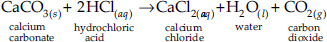Q 5.

Why do HCl, HNO3, etc. show acidic characters in aqueous solutions while solutions of compounds like alcohol and glucose do not show acidic character?

SOLUTION:

HCl and HNO3 produce H+(aq) ions in aqueous solution, which is responsible for their acidic character.
HCl(aq) → H+(aq) + Cl(aq)
HNO3(aq) → H+(aq) + NO3(aq)
Alcohol (C2H5OH) and glucose (C6H12O6) are covalent compounds and they do not undergo dissociation in aqueous solution. This is evident from the fact that their aqueous solutions do not conduct electricity. Hence, the aqueous solutions of alcohol and glucose do not show acidic character even though they contain hydrogen atoms.

Q 6.

Why does an aqueous solution of acid conduct electricity?

SOLUTION:

An aqueous solution of acid produces ions and therefore, it conducts electricity. The electric current is carried through the solution by ions.

Q 7.

Why does dry HCl gas not change the colour of the dry litmus paper?

SOLUTION:

Dry HCl gas does not show acidic character due to absence of H+ ions and therefore, does not change the colour of the dry litmus paper.

Q 8.

While diluting an acid, why is it recommended that the acid should be added to water and not water to the acid?

SOLUTION:

Dilution of a concentrated acid, particularly concentrated sulphuric acid, is a highly exothermic reaction. When water is added to a concentrated acid, the heat liberated is so large that the solution starts almost boiling. This may cause spurting of the hot acid solution and harm the person. Excessive local heating may even break the glass container. That is why concentrated acids are diluted by slowly adding concentrated acid into water with constant stirring and not by adding water to the acid.

Q 9.

How is the concentration of hydronium ions (H3O+) affected when a solution of an acid is diluted?

SOLUTION:

When the solution of acid is diluted the H+ ions are released from the acid to combine with H2O and H3O+ ions is increased.

Q 10.

How is the concentration of hydroxide ions (OH) affected when excess base is dissolved in a solution of sodium hydroxide?

SOLUTION:

The OH concentration increases with the concentration of NaOH and reaches a limiting value.

Q 11.

You have two solutions, A and B. The pH of solution A is 6 and pH of solution B is 8. Which solution has more hydrogen ion concentration? Which of this is acidic and which one is basic?

SOLUTION:

pH of solution A = 6.
∴ H+ ion concentration = 10–6 M.
pH of solution B = 8.
∴ H+ ion concentration = 10–8 M.
As 10–6 > 10–8, hence solution A has more H+ ion concentration.
A solution with pH < 7 is acidic. Hence, solution A is acidic.
A solution with pH > 7 is basic. Hence, solution B is basic.

Q 12.

What effect does the concentration of H+(aq) ions have on the nature of the solution?

SOLUTION:

If a solution has higher concentration of H+ ions it is more acidic in nature.

Q 13.

Do basic solutions also have H+(aq.) ions? If yes, then why are these basic?

SOLUTION:

Basic solutions also have H+ ions in addition to OH ions. They are basic because in these solutions, OH ion concentration is greater than H+ ion concentration.
In a basic solution, H+(aq) concentration is much lower than the OH(aq) concentration.

Q 14.

Under what soil condition do you think a farmer would treat the soil of his fields with quick lime (calcium oxide) or slaked lime (calcium hydroxide) or chalk (calcium carbonate)?

SOLUTION:

If the soil condition is more acidic than optimum conditions.

Q 15.

What is the common name of the compound CaOCl2?

SOLUTION:

Bleaching powder.

Q 16.

Name the substance which on treatment with chlorine yields bleaching powder.

SOLUTION:

Dry slaked lime, Ca(OH)2.

Q 17.

Name the sodium compound which is used for softening hard water.

SOLUTION:

Sodium carbonate (washing powder).

Q 18.

What will happen if a solution of sodium hydrogen carbonate is heated? Give the equation of the reaction involved.

SOLUTION:

When a solution of sodium hydrogen carbonate is heated it gives sodium carbonate, carbon dioxide and water.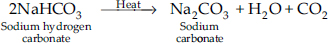Q 19.

Write an equation to show the reaction between plaster of Paris and water.

SOLUTION:

It forms gypsum.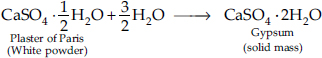Q 20.

A solution turns red litmus blue, its pH is likely to be

 A. 1 B. 4 C. 5 D. 10

SOLUTION:

This is because the solution is basic.

Q 21.

A solution reacts with crushed egg-shells to give a gas that turns lime-water milky. The solution contains

 A. NaCl B. HCl C. LiCl D. KCl

SOLUTION:

Egg-shells contain calcium carbonate. Calcium carbonate reacts with HCl to give out CO2 gas, which turns lime water milky.

Q 22.

10 mL of a solution of NaOH is found to be completely neutralised by 8 mL of a given solution of HCl. If we take 20 mL of the same solution of NaOH, the amount of HCl solution (the same solution as before) required to neutralise it will be

 A. 4 mL B. 8 mL C. 12 mL D. 16 mL

SOLUTION:

When the volume of NaOH solution is doubled, the amount of HCl solution required to neutralise NaOH will also be doubled.
20 mL of NaOH = 2 × 8 mL of HCl = 16 mL of HCl.

Q 23.

Which one of the following types of medicines is used for treating indigestion?

 A. Antibiotic B. Analgesic C. Antacid D. Antiseptic

SOLUTION:

The indigestion is due to excess of acid produced in the stomach.The medicine used to neutralise it is called antacid.

Q 24.

Write word equations and then balanced equations for the reaction, taking place when:

 A. Dilute sulphuric acid reacts with zinc granules B. Dilute hydrochloric acid reacts with magnesium ribbon C. Dilute sulphuric acid reacts with aluminium powder D. Dilute hydrochloric acid reacts with iron fillings.

SOLUTION: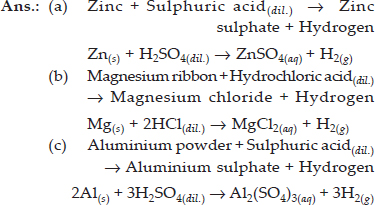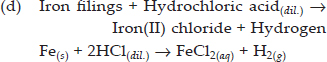Q 25.

Compounds such as alcohol and glucose also contain hydrogen but are not categorised as acids. Describe an activity to prove it.

SOLUTION:

Activity : To show that alcohols and glucose are not acids.
Materials required : Dilute solution of ethanol and glucose.
Apparatus required : Beaker (1), carbon electrodes (2), dry cells (2), bulb 1.5 V (1), key (1).
Procedure : Take a beaker and place two carbon electrodes into it. Connect the electrodes to a battery bulb through a key and a dry cell. Pour ethanol into the beaker and press the key. See, if the bulb glows. Bulb does not glow.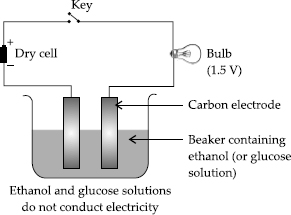Repeat similar experiment with glucose solution. Record your observations.
Observation : It is observed that the bulb does not glow with both the solutions.
Conclusion : The solutions of glucose and ethanol are non-conductors of electricity.
Explanation : Ethanol and glucose contain hydrogen in their molecules and do not produce H+ ions in solutions. Hence these are not categorised as acids.

Q 26.

Distilled water does not conduct electricity, whereas rain water does. Why?

SOLUTION:

Distilled water is pure and it does not form ions. Whereas rain water contains impurities in it like acid which contains ions and release them when dissolved in water. Hence, no electricity is conducted by distilled water but ions are there in rain water so electricity is conducted.

Q 27.

Why acids do not show acidic behaviour in the absence of water?

SOLUTION:

Acids ionise only in the presence of water to give ions.
HCl + H2O → H3O+ + Cl
However, in the absence of water, acids do not ionise to give H3O+ ions and therefore, do not behave as acids.

Q 28.

Five solutions A, B, C, D and E when tested with universal indicator showed pH as 4, 1, 11, 7 and 9 respectively. Which solution is:
a. neutral?
b. strongly alkaline?
c. strongly acidic?
d. weakly acidic?
e. weakly alkaline?
f. Arrange the pH in increasing order of hydrogen-ion concentration.

SOLUTION:

Solution D is neutral (pH = 7).
(b) Solution C is strongly alkaline (pH = 11).
(c) Solution B is strongly acidic (pH = 1).
(d) Solution A is weakly acidic (pH = 4).
(e) Solution E is weakly alkaline (pH = 9).
(f) pH in the increasing order of hydrogen ion concentration: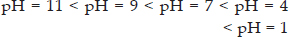Q 29.

Equal lengths of magnesium ribbons are taken in test tubes A and B. Hydrochloric acid (HCl) is added to test tube A, while acetic acid (CH3COOH) is added to test tube B. In which test tube will the fizzing occur more vigorously and why?

SOLUTION:

In test tube A hydrochloric acid is present which is a strong acid as compared to acetic acid present in test tube B.
The fizzing occurs more vigorously in test tube A as HCl is strong acid and dissociates completely into H+ and Cl ions.

Q 30.

Fresh milk has a pH of 6. How do you think the pH will change as it turns into curd?

SOLUTION:

Curd is sour in taste and acidic. Therefore, its pH will decrease from pH of 6 to a lower value.

Q 31.

A milkman adds a very small amount of baking soda to fresh milk.
a. Why does he shift the pH of the fresh milk from 6 to slightly alkaline?
b. Why does this milk take a long time to set as curd?

SOLUTION:

(a) The milkman adds baking soda to milk so that the milk becomes slightly alkaline. Thus, milk will not be converted to curd readily.
(b) This will take a longer time to set to curd because it is alkaline and takes longer time for bacteria to make it acidic.

Q 32.

Plaster of Paris should be stored in a moisture proof container. Explain why?

SOLUTION:

Plaster of Paris in contact with moisture (water) changes to solid hard mass, gypsum. Therefore, it gets wasted. Hence it should be stored in moisture proof containers.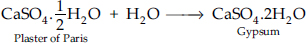Q 33.

What is neutralisation reaction? Give two examples.

SOLUTION:

The reaction of an acid and a base to form salt and water is a neutralisation reaction. For example,
(i) HCl + NaOH → NaCl + H2O
(ii) H2SO4 + 2KOH → K2SO4 + 2H2O

Q 34.

Give two important uses of washing soda and baking soda.

SOLUTION:

Washing Soda
(1) It is used for softening of hard water.
(2) It is used for the manufacture of soap, glass, paper, borax, caustic soda, etc.
Baking soda
(1) Baking soda is mainly used in the preparation of baking powder.Baking powder contains sodium hydrogen carbonate and tartaric acid.
(2) Baking soda is used in medicines as an antacid.It is used in medicines to remove acidity of the stomach.